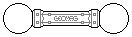Web textodigital.com
Pseudogeodesic spheres
We call pseudogeodesic sphere to a closed solid made of 12 pentagons, and 30 hexagons. All 42 polygons are regular, although the hexagons cannot be really planar. The first of the following pages explains how to get the object from a regular dodecahedron to double scale, in the second and third pages we build the sphere to scales 2 and 3, and in the fourth one we build a fragment of the sphere to scale 4: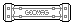As each hexagon is made of 6 triangles, and each pentagon is made of 5 triangles, the pseudogeodesic sphere is made of 30 × 6 + 12 × 5 = 240 triangles. Each of the triangles uses 3 rods (so, 240 × 3 = 720 rods), but, as each rod belongs in two triangles simultaneously, we would be counting them twice; the right figure is 720 / 2 = 360 rods.
Similarly, each triangle has three vertices (so, 240 × 3 = 720 balls). Most vertices are shared by 6 triangles, but the 12 central vertices in the pentagons are shared by only 5 triangles. So, counting the total number of balls is not as easy as dividing 720 by 6. It can be done in this way: each one of the 12 central vertices in the pentagons is shared by 5 triangles, so they are adding 12 × 5 = 60 to the total of 720; if we substract them, the 720  60 = 660 remaining balls have been counted 6 times indeed, as every remaining vertex is shared by 6 triangles, so they really are 660 / 6 = 110 balls. Adding them to the previous 12 gives a total of 110 + 12 = 122 balls.
We could have found the same number using the polyhedral formula studied at school (V stands for vertices, E for edges, and F for faces): V = E  F + 2, that is, V = 360  240 + 2 = 122 balls.
In order to build a pseudogeodesic sphere to a different scale, we can substitute a new triangle made to the desired scale for each single triangle of the object. Here we show as the equilateral triangles to scales 2, 3, 4, and 5 are made of 4, 9, 16, and 25 triangles, respectively: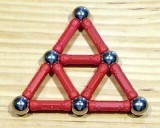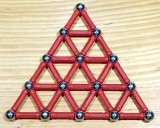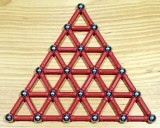An equilateral triangle to scale n is made of n2 single triangles. To compute the piece quantities to scale n, it's enough to multiply the number of triangles (240) by n2, and to repeat the previous computation (to any scale, the number of vertices shared by five triangles is 12). The following table gives several computed results, and the general formulas:
 scale scale2 triangles rods balls 1 1 240 360 122 2 4 960 1440 482 3 9 2160 3240 1082 4 16 3840 5760 1922 5 25 6000 9000 3002 6 36 8640 12960 4322 7 49 11760 17640 5882 8 64 15360 23040 7682 ... ... ... ... ... n n2 240 × n2 360 × n2 120 × n2 + 2